## Indicators

•Cited by SciELO
•Access statistics

•Cited by Google
•Similars in SciELO
•Similars in Google

## Print version ISSN 1794-1237On-line version ISSN 2463-0950

### Rev.EIA.Esc.Ing.Antioq  no.10 Envigado July/Dec. 2008

CONDITIONAL VOLATILITY OF COLOMBIAN GOVERNMENTAL FIXED INCOME SECURITIES AS A PREDICTOR OF SHORT-TERM RETURNS

VOLATILIDAD CONDICIONAL DE LOS TÍTULOS DE RENTA FIJA DEL GOBIERNO COLOMBIANO COMO PREDICTOR DE LOS RETORNOS DE CORTO PLAZO

Javier O. Pantoja*

* PhD student in Management, major finance HEC University Montreal QC. Assistant Professor in Business School in Eafit University. javier-orlando.pantoja-robayo@hec.ca; jpantoja@eafit.edu.co

Discusión abierta hasta junio de 2009

ABSTRACT

According to literature, the long-maturity forward rates have information about the structure of the expected short-term returns. This paper finds that the conditional volatility factor also has information for predicting the term premium in the six-month expected returns with different maturities. That is, including conditional volatility allows capturing a risk factor consistent with the agent's expectations. A slow mean-reverting process is also found across different maturities, which is the case of the governmental fixed income securities. In fact, the power of forecasting changes from a six-month to a three-year forward period, at six-month steps according to the mean-reverting tendency which also implied that its predictive power improves at longer forecasting horizons. On the other hand, it presents evidence about the Colombian markets crash in May 2006, which generated special conditions that impacted the market's behavior and the agent's risk tolerance.

KEY WORDS: conditional volatility; short term return structure; forward rates; GARCH models; term premium; governmental fixed income securities.

RESUMEN

De acuerdo con la literatura las tasas de interés futuras de largo plazo ofrecen información relevante que las convierte en un buen predictor de la estructura de retornos de corto plazo. Este artículo encuentra que el factor de volatilidad condicional posee información para predecir la prima a plazo con intervalos de seis meses para diferentes vencimientos. En otras palabras, incluir la volatilidad condicional como factor permite captar las señales de riesgo en consonancia con las expectativas de los agentes. Se evidencia, además, un proceso lento de reversión a la media para diferentes vencimientos en los títulos gubernamentales, lo cual incide sobre la capacidad de predicción, que en este caso muestra ser mayor para vencimientos más tardíos. Por otra parte, se presenta evidencia sobre la caída del mercado colombiano en mayo de 2006, hecho que generó condiciones especiales de operación, además de impactar sobre la percepción de riesgo de los agentes.

PALABRAS CLAVE: volatilidad condicional; estructura de retornos de corto plazo; tasas de interés futuras; modelos GARCH; prima de riesgo; títulos gubernamentales de renta fija.

1. INTRODUCTION

The dynamic of the short-term interest rate has fundamental information which can be used for valuating processes and for pricing fixed income securities and their extension as well as derivatives that use them as underlying assets. Several authors have already concluded papers where two different approaches are presented to estimate the structure of the expected short-term returns; the standard noarbitrage approach1 and the equilibrium model2. The first finds the term-structure of interest rate model, the second basic approach finds the process that the term-structure follows. In this case, the second basic approach is appropriate with conditions of the fixed income security markets. This paper finds that expected short-term returns depend on factors such as variations in expected spot-rates, which are captured by conditional volatility factor and also, variations in current forward rates. So the main testable theory such as the Pure Expectation Hypothesis (PEH) sustains that bond yields are given by the unbiased expectations of future-short-term interest rates given by the forward rates, plus a non-negative risk premium; it gives support for modeling the term premium in the six-month-expected returns with different maturities. Therefore, this paper studies the information in conditional volatility and forward rates about the current expected returns for semiannually Colombian Treasury maturities to three years.

In addition, the Colombian markets had crashed in May 2006, which generated special conditions that impacted the market's behavior and presented a structural change, where series before and after show different pattern trends and also different considerations about the agent's risk tolerance. In fact, the crash produced a structural change3 which generated difficulty to demonstrate stationary of the series. We had split data into two series. Firstly, daily data was taken from August 1, 2002 to May 5, 2006. Secondly, daily time-series data was taken from May 6, 2006 to February 7, 2008; in Figure 1, structural change can be seen. The reason why data series were split was to explore differences in predictability of short-term returns from the conditional volatility factor and current forward rates for each scenario, without structural change effect. Both, data series before and after the crash were used for predicting expected short-term returns.

The results of the paper on expected returns produce reliable inferences about the structure of expected returns for maturities beyond a year. Using cross-sectional time-series general least square (GLS) regression approach, this paper extends Fama and Bliss (1987) model of the expected short-term return as a function of the corresponding forward rate, towards the use of one more factor which is the conditional volatility of Colombian Treasury Bond (T-Bonds) returns. On the other hand, this paper finds significant evidence about the forecast power of the factors used such as forward rates and conditional volatility of the Colombian T-bonds. Evidence suggests that forward rates and volatility of T-bonds can forecast future short-term returns, in contrast with previous tests that found little evidence of the power of forecast as a possible tool4. It was also found that the power of forecast increases at a time in which the forecast horizon is extended because the series show slow mean-reverting tendency. Furthermore, its predictive power improves at longer forecast horizons. In fact, hypothesis that interest rates are mean reverting tendency was researched by several authors5. In order to find the mean-reverting condition in the series, augmented Dickey-Fuller (1979), Phillips- Perron (1988) and Kwiatkowski-Phillips-Schmidt-Shin (1992) unit-root statistic tests for detrained data were applied, in which trend effects caused by sensor drift were removed. Therefore, these tests have essentially researched whether the predictability condition is presented in our explicative variables. Results showed that the series are stationary. The tests results are shown in Appendix 1 for both scenarios: before and after the crash.

In order to build a set of explicative variables, conditional volatility was modeled using the generalized autoregressive conditional heteroskedasticity volatility modeling –GARCH model– (Bollerslev, 1986), which is specified as: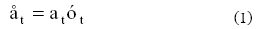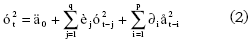The "p" order is associated with temporal dependence upon the time series variance with stochastic square collisions that took place "p" periods ago. The "q" order is associated with temporal dependence upon the same time-series in "t" period and the value given in last "q" periods. The error term is independent from mean zero and constant variance. In addition, we used GARCH model (Engle, 1982), via maximum likelihood where distributional assumptions were: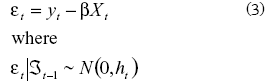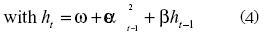Therefore, GARCH (p, q) model with t-distribution and p=1 and q=2 was used. In the next step, the expected term-premium return using cross sectional GLS regression model was used to obtain the expected term-premium return for different maturities and with two series: before and after the crash. Those series have three and two years forward at six-month steps. The plots of the interest rate volatility estimated by GARCH (1, 2) model for pre-crash period are shown in Appendix 3 and 4, those graphs and the model were constructed using MATrix LABoratory program –MATLAB.Figure 1shows the colombian treasury bonds for maturities from a six- mont to a three-year forward period, at six-month steps in daily pattern, and also it shows the moment of the Colombian markets crash in may 2006.

The data for this paper consists of the daily trading rates of the Colombian Bonds prices in Colombian Pesos (COP) of the National Electronic Market of the Colombian Security Exchange, separated into two series; the first, from August 1, 2002 to May 5, 2006, and the second, from May 6, 2006 to February 7, 2008; both series are expressed in a daily pattern. The instantaneous forward rate for each maturity horizon can be obtained by means of a differential equation.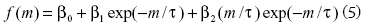Where t is a positive constant and β0, β1, β2, β3 are the parameters to be estimated. The next equation was used to obtain the instantaneous forward rate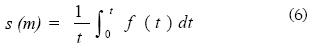Finally, the followin equation is obtained if we derivate previous equation (Nelson and Siegel 1987)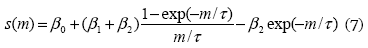Where β0, β1, β2, β3 and τ are the parameters and "m" represents the maturity horizons. We used series and parameters to estimate zero coupon rates and forward rates for maturities in each trading day. Parameters β0, β1, β2, β3 and τ were obtained using the daily trading rates, by means of bootstrapping technique. Based on this method, spot and forward rates can be approximated to a constant in the short and long term.

This paper presents evidence about the term premium in the six-month expected returns with different maturities on governmental fixed income securities and includes significant conditions about agents expectative that are relevant to the Colombian markets conditions, whose results are perceptible in current bond prices and interest rates. Fama and Bliss (1987) found that current forward rates on one-to 5-year U.S. Treasury Bonds have information about the current term structure of 1-year expected return on the bonds and explained that 1-year forward rates forecast changes in the 1-year interest rate 2-to 4-year ahead, and they focused on the increasing forecast power with the forecast horizon. Brenner (1996) presented a relationship between interest rate and bond prices volatility. Dankenbring (1996) found that, in the German economy, volatility of interest rate depends as well on the stochastic perturbations associated with new information. Zuñiga (1999) found that GARCH model brought best information to explain volatility behavior of the short-term interest rate. Other papers focused on the interest rate estimation including Ball and Torous (1996), Longstaff and Schwartz (1992), Mashayekh (1996). This paper shows that the conditional volatility factor also has information in Colombian market for predicting the term premium in the six-month expected returns with different maturities. In Section 2, empirical work is described, and Section 3 presents a conclusion.

2. EMPIRICAL WORK

In order to clarify the following elaborations, a definition of the variables is provided. The term premium return (Tpr) on a (t – Sm) discount bond bought at time t0 and sold at t0 + t – 1 where t takes 2-Sm, 3-Sm, 4-Sm, 5-Sm and 6-Sm values and the bond was sold when it has Six-month to maturity. Furthermore, the holding return is defined as follow: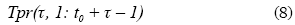And the six-month forward rates for the periods from t0 + τ – 1 t0 t0 + τ is defined as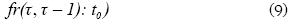And the six-month forward rates can be expressed like a difference of rates as follows: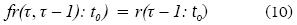Since r(τ: t0 ) = –ln p(τ: t0) then the next relation can be established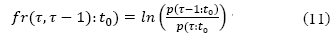The current price at time t0 of the τ-1 discount bond that pays \$1 at maturity is the present value of the expected six-month returns on the bond.The next expression shows the current bond price.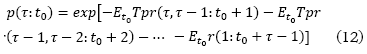Hence,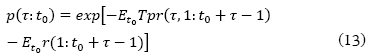The last equations were obtained by definition of returns, which express that the price contains forecast of equilibrium expected return (fama and Bliss, 1987). Under the assumption that forward rates reasonably forescast the current six-month spot rate r (1: t0+ τ - 1) to observe at time t0+ τ - 1 moreover, fr ( τ, τ - 1: t0 has rational information about r(1: t0+ τ - 1). If equation 13 is combined witch equation 11 and subtracting a six-month spot rate r (1: t0),the next relation could be established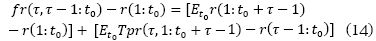The forward spot spread could be established as fr ( τ, τ - 1: t0) - r (1: t0). Also, it can be found that realized returns are the same that the expected value of the future six-month return. So that the next expression is produced from equiation 14.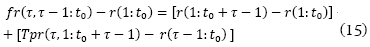According to the pure expectation hypothesis (Fisher, 1986), forward rates contain forecast of the market's expectations of future short- term rates, which is expressed in the next equation: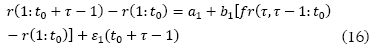If we combined equations 15 and 16, the next relation could be established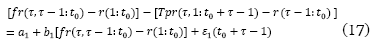Hence,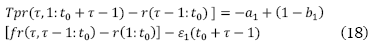Including the new factor and organizing the variables, in order to capture the volatility effect, the next model could be built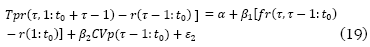Estimation of the expected term premium return is based on risk measures that capture unexpected changes on spot rates and risk preferences, in order to capture significant effects in structure of the spot rates, so that expected changes in the expected short-term return from day t through day t+1 can be estimated. Using information of the daily trading rates of the Colombian Bonds prices in COP from the National Electronic Market of the Colombian Security Exchange, from August 1, 2002 to May 5, 2008 for the first series, and from May 6, 2006 to February 7, 2008 for the second series, registered day by day, the term-premium return obtained using equation 8, the forward rates from equation 9, and the conditional volatility obtained from GARCH (1, 2) model could be obtained. Those variables were used to estimate and establish the first one, such as endogenous variable was included a new set of variables.The realized term premium return expressed by Tpr(τ, 1: t0 + τ – 1) – r(τ, 1: t0 ), where t represents different maturities from one to three years for the first series and one to two years for the second series, both at six-month steps. The t0 represents the actual date or zero in the time scale and 1 represents one six-month period. The second, the six-month forward spot spread expressed by fr(τ, τ – : t0 ) – r(τ, : t0 ) or forward spot spread from τ – 1 to τ. Finally, the conditional volatility expressed by CVp(τ – : t0 ) or conditional volatility from t0 to τ – and the spot rate denoted by r(τ, : t0 ) was used.In that case, the spot rate obtained from a six-month Treasury bill as a variable was used to establish an econometric condition. These explanatory variables permit to forecast expected short-term return, using cross-sectional time-series GLS regression. Appendix 2 presents statistics for the risk factors statistically significant.

Evidence shows that volatility pattern on interest rates depends on the stochastic perturbations associated with risk preferences of the agents, such as that illustrated in Figure 2.

Besides, evidence shows that coefficients β1 and β2 are greater than zero, which means that forward spot spread and conditional volatility factors observed at time t0 have power to forecast changes on the six-month spot rate (τ –1 ) periods ahead. Also, our evidence shows that firstly slope in forward spot spread β1 for different maturities tends to be constant throughout time. Slope β1 shows values around 0.60, which implies that it does not improve at a longer forecast horizon due to future spot rate r(1: t0 + τ – 1) in equation 16, and it is calculated from the six-month Treasury bills at time (t0 + τ – 1) which generates measurement errors in the prices valuated at time t0 and bias in forward spot spread is introduced and the forecast power in the forward rate is difficult to consider. Secondly, the conditional volatility factor has power to forecast the term premium (τ – 1) six-month return because, surprisingly, the predictive power of forecasting in the conditional volatility increases at a longer forecast horizon on the pre-crash period according to agent's expectative. Slope β2 goes with values from –1.402295 to 12.255030, which means that agents show risk tolerance in that economic period and they prefer short-term to invest. On the post-crash period, the first periods show the same behavior, slope b2 goes with values from 6.282145 to 12.534680 and 9.335782 in the end period. Appendix 2 and 3 show that, for maturities τ at six-month steps, the impact of conditional volatility in each period is greater than previous maturities, which means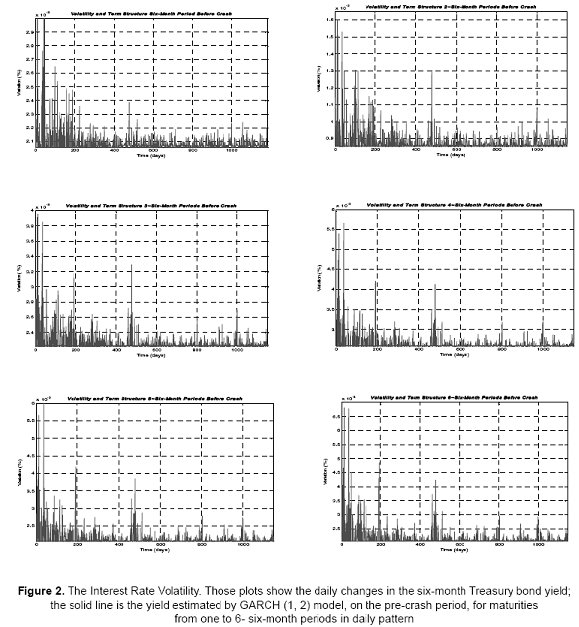that expected return increases with the maturity according to the liquidity preference hypothesis (Hicks, 1939 and Kessel, 1965). If the liquidity premium α = – a is considered, it could be observed (for example) that the premium increases monotonically throughout the maturity rising from –0.0436289 to 0.4556571 in pre-crash period.

3. CONCLUSION

The estimates of the term premium return, using cross-sectional GLS regression, demonstrate that, due to conditional volatility and slow meanreverting tendency, the expected term premium return changes throughout time and the power of forecast of the conditional volatility increases at longer forecast horizon which is evident on the pre-crash period, where slopes of the volatility factor goes from –1.402295 to 12.255030 across different maturities such as a sign of the agents expectative. Moreover, evidence shows that agents prefer shorter term bonds to invest than short or long term bonds. Besides, the post-crash period shows the same pattern in the first and second maturity but decreasing at the end of the maturity bond. According to the liquidity preference hypothesis, this model is equivalent to the liquidity premium constant α = – a, which increases monotonically throughout the maturity, taking into account that agents prefer shorter term bonds to invest. On the other hand, based on the conditional volatility factor, longer term bonds are riskier than shorter bonds. Moreover, term premium return is higher for long-term maturities.

1 This approach is followed by Ho and Lee (1986), Derman and Toy (1990), Hull and White (1990), among others.

2 This approach is followed by Merton (1973), Vasicek (1977), Brennan and Schwartz (1980), Cox, Ingersoll and Ross (1985), for one factor, and Brennan and Schwartz (1979), Longstaff and Schwartz (1992), among others.

3 Using CUSUM test with OLS residuals, Chow test and Wald test statistics, we proved presence of structural change in the specific date.

4 Michel Hamburger and E. N. Platt (1975) and Robert J. Shiller et al. (1983) concluded that results on the forecast power of forward rates do not exist. So did Fama (1984a), confirming that forward rates forecasts of near-term changes in interest rate are poor.

5 Nelson and Plosser (1982) and Fama and Gibbons (1984), among others.

BIBLIOGRAPHY

Ball, C. A. and Torous, W. N. (1996). Unit roots and estimation of interest rate dynamics. The Journal of Empirical Finance. Vol. 3, 215-238.        [ Links ]

Bollerslev, T. (1986). Generalized autoregressive conditional hereroskedasticity. Journal of Econometrics. Vol. 31, 307-327.        [ Links ]

Cox, J. C., Ingersoll, J. E. and Ross, S. A. (1985). A new theory of the term structure of interest rate. Econometrica. Vol. 53, 385-407.        [ Links ]

Dankenbring, H. (1998). Volatility estimates of the short term interest rate with an application to German data. Humboldt- and Free University Berlin, working paper.        [ Links ]

Dickey, D. and Fuller, W. (1979). Distribution of the estimates for autoregressive time series with unit root. Journal of American Statistical Association, 74, 427-431.        [ Links ]

Engle, R. (1982). Autoregressive conditional heteroskedasticity with estimates of the variance of United Kingdom inflation. Econometrica. Vol. 50, 987-1008.        [ Links ]

Fama, E. F. (1984). "The information in the term structure", Journal of Financial Economics. Vol. 13, 509-528.        [ Links ]

Fama, E. F. and Bliss, R. (1987). The information in longmaturity forward rates. The American Economic Review. Vol. 77, No. 4, 680-692.        [ Links ]

Fisher, I. (1896). Appreciation and interest. AEA Publications. Vol. 11 (3), 331-442.        [ Links ]

Hicks, J. (1939). Value and capital. Oxford University Press.        [ Links ]

Ho, T. and Lee, S.-B. (1986). Term structure movements and pricing interest rate contingent claims. The Journal of Finance. Vol. 41, No. 5, 1011-1029.        [ Links ]

Hull, J. and White, A. (1990). Valuing derivative securities using the explicit finite difference method. Journal of Financial and Quantitative Analysis. Vol. 25 (1) 87-100.        [ Links ]

Kwiatkowski, D., Phillips, P., Schmidt, P. and Shin, Y. (1992). Testing the null hypothesis of stationary against the alternative of a unit root. Journal of Econometrics. Vol. 54, 159-178.        [ Links ]

Longstaff, F. A. and Schwartz, E. S. (1992). Interest rate volatility and the term structure: a two factor general equilibrium model. Journal of Finance. Vol. 47, No. 4, 1259-1282.        [ Links ]

Merton, R. C. (1973). Theory of rational pricing. Bell Journal of Economics and Management Science. Vol. 4, 141-183.        [ Links ]

Nelson, C. R. and Siegel, A. F. (1987). Parsimonious modeling of yield curves. The Journal of Business. Vol. 60, No. 4, 473-489.        [ Links ]

Phillips, P. and Perron, P. (1988). Testing for a unit roots in time series regression. Journal of Econometrics. Vol. 33, 335-346.        [ Links ]

Vasicek, O. (1977). An equilibrium characterization of the term structure. Journal of Financial Economics, Vol. 5, 177-188.        [ Links ]

APPENDIX

Appendix 1: Unit Root Test Statistical

This table presents summary statistics for unit-root test (augmented Dickey-Fuller, Phillips-Perron and Kwiatkowski-Phillips-Schmidt-Shin tests statistic). Critical values for augmented Dickey-Fuller and Phillips- Perron tests statistic test statistic (-1.941118) is used at the 95% level, asymptotic critical value for Kwiatkowski- Phillips-Schmidt-Shin test statistic is 0.463 at the 95% level

Appendix 2: Results from Regressions of Expected Term Premium Return

This table presents summary statistics from cross-sectional time-series GLS regression model using the time series of the daily trading rates of the Colombian Bonds prices in COP from the National Electronic Market of the Colombian Security Exchange, from August 1, 2002 to February 7, 2008, registered day by day; the spot rates and the six-month forward rates; two variables were included; firstly, the six-month forward spot spread expressed by fr(τ, τ – 1: t0 ) – r(1: t0 ) or forward spot spread from τ – 1 to τ; secondly, the conditional volatility expressed by CVp(τ – 1: t0) or conditional volatility from t0 to τ– 180 – days; the spot rate was also used, denote by r(1: t0)

Appendix 3. Fitter and realized term premium return before crash

This plot shows the percentage term-premium return before crash. Both, realized and predicted values, were obtained from cross sectional GLS regression from six month to three years forward, at six-month steps, for maturities at six-month, 2-six-month, 3-six-month, 4-six-month, 5-six-month, 6-six-month in each trading day of the National Electronic Market of the Colombian Security Exchange, from August 1, 2002 to May 5, 2006

Appendix 4. Fitted and realized term premium return after crash

This plot shows the percentage term-premium return after crash. Both, realized and predicted values, were obtained from cross sectional GLS regression from six month to two years forward, at six-month steps, for maturities at six-month, 2-six-month, 3-six-month, 4-six-month, in each trading day of the National Electronic Market of the Colombian Security Exchange, from May 6, 2006 to February 7, 2008All the contents of this journal, except where otherwise noted, is licensed under a Creative Commons Attribution License